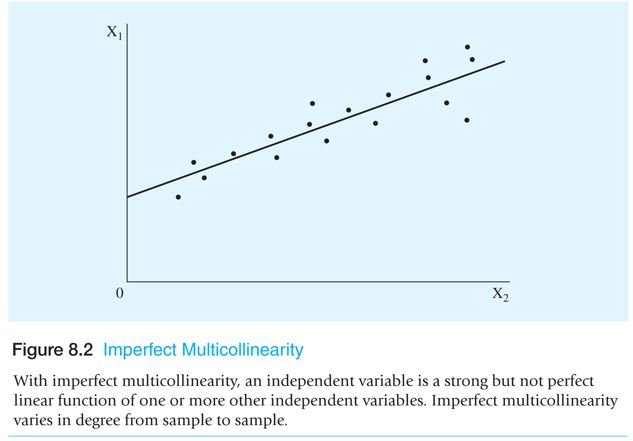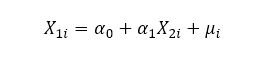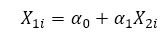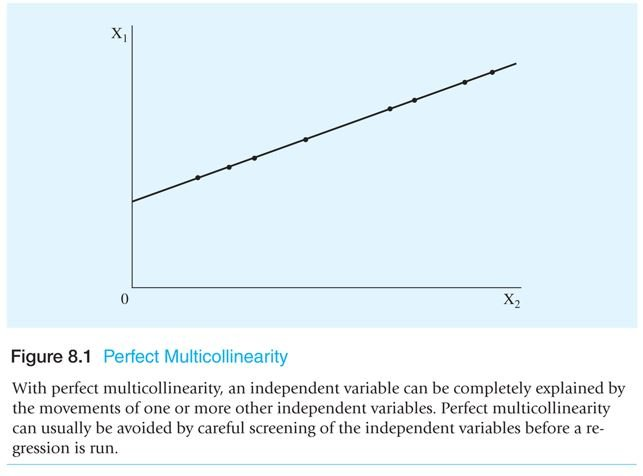Select Page

## Imperfect multicollinearity

• With imperfect multicollinearity, an independent variable has a strong but not perfect linear function of one or more independent variables.
• This also means that there are also variables in the model that effects the independent variable.
• In other words If there are two independent vairables that are related to each other. Yet there are also other vairables out of the model that effects one of the independent variable which means that there is no perfect linear function between the two only. Thus, the inlcuson of a stochastic term in the model shows that the existence of other vairables are also effecting the regressors.
• Imperfect multicolinearity varies in degree to degree according to the sample size.
• The presence of the error term dilutes the relstionship between the independent vairables.

Mathematically and Graphically:The equations tell us that there might be a relationship between X1 and X2 but it does not explain that X1 is to be completely explained by X2; there is a possibility of unexplained variations as well, in the form of the stochastic error term.

## Perfect collinearity

The word perfect explains that a variation in one explanatory variable can be completely explained by movements in another explanatory variable.

A perfect relationship between two variables would be somewhat like:Where:  is the constant and the Xs are the independent variables. Since there is no error term in the equation, it can be stated that x1 can be exactly explained by X2.Perfect multicollinearity is easy to discover compared to imperfect multicollinearity.To detect perfect multicollinearity:

1. If one variable is equal to the multiple of another variable
2. If one variable is derived by adding the constant to another variable
3. If a variable equals the sum of two other variables.

OLS, in this case, is not capable of generating or estimating outcomes. Because multicollinearity ruins the ability to estimate the coefficients because the two variables cannot be distinguished.

Shares